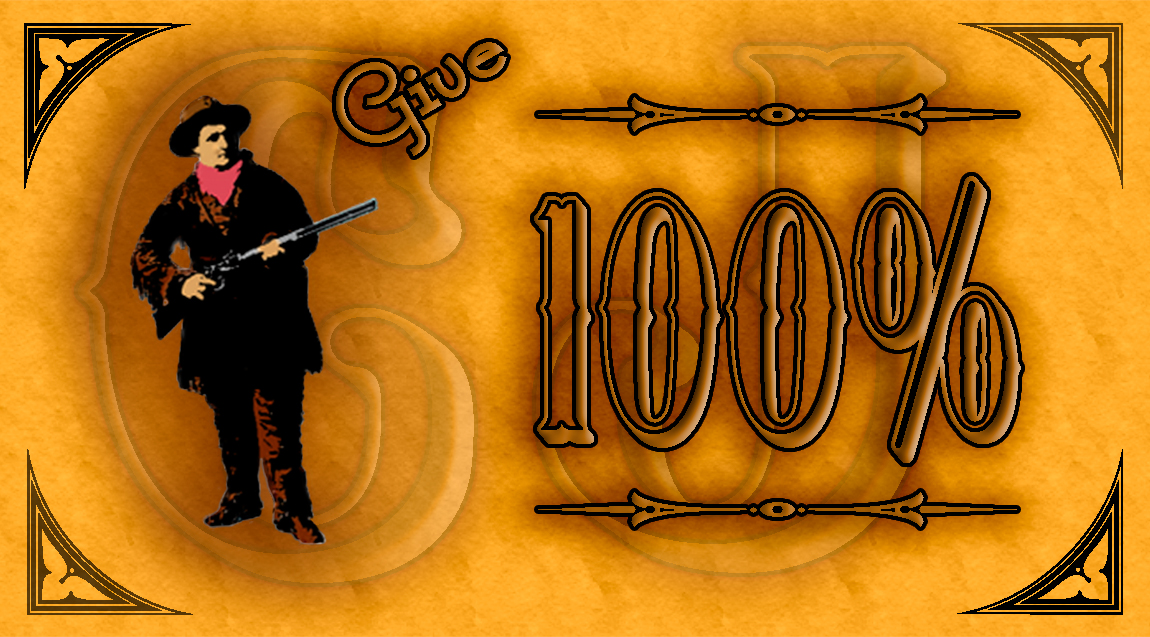# Give 100%Ever wonder about those people who say they are giving more than 100%? We have all been to those meetings where someone wants you to give over 100%.

What makes up 100% in life?

Here’s a little mathematical formula

If:
A B C D E F G H I J K L M N O P Q R
S T U V W X Y Z

Is represented as:
1 2 3 4 5 6 7 8 9 10 11 12 13 14 15
16 17 18 19 20 21 22 23 24 25 26.

Then:

H-A-R-D-W-O-R-K
8+1+18+4+23+15+18+11 = 98%

And

K-N-O-W-L-E-D-G-E
11+14+15+23+12+5+4+7+5 = 96%

But ,

A-T-T-I-T-U-D-E
1+20+20+9+20+21+4+5 =100%

And,

B-U-L-L-S-H-I-T
2+21+12+12+19+8+9+20 = 103%

Just look how far ass kissing will take you.

A-S-S-K-I-S-S-I-N-G
1+19+19+11+9+19+19+9+14+7 = 118%

So, one can conclude with mathematical certainty, that while hard work and knowledge will get you close, and attitude will get you there. It’s the Bullshit and Ass Kissing that will put you over the top.

Now you know why Politicians are
where they are!

Calamity Jane